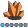qkaiwei
Calcite | Level 5

## Optmodel problem

A question about constraint statement of optmodel  procedure, sum of elements in array x must be less or equal to 100, and number of elements greater than 0 must be less or equal to 3, I don't know how to write this properly.

Thanks!

proc optmodel:

set<number> dates=1..10;

var x{dates} >=0 init 0;

......

con c1: sum{i in dates} x[i] <=100;

con c2: sum{i in dates}  /*(x[i]>0)*/  <= 3;

quit;

## Re: Optmodel problem

Introduce a binary variable y[i] that is 1 if x[i] > 0:

``````   var x{dates} >=0 init 0 <= 100;
con c1: sum{i in dates} x[i] <=100;
var y{dates} binary;
con c2: sum{i in dates} y[i] <= 3;
con c3 {i in dates}: x[i] <= x[i].ub * y[i];
``````
Discussion stats